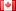# Revision history [back]

### riemann roch basis

i have a question with this code (i speak little english)

F.<w>=GF(4,'w') R.<x,y,z> = ProjectiveSpace(F,2) C = Curve(X^2Y + wY^2Z+ w^2Z^2*X) print C.genus() pts = C.rational_points() print pts print pts D = C.divisor([ (2, pts),(1, pts) ]) print D print C.riemann_roch_basis(D)

result:

(1 : w : 1) (w : 1 : 1) 2(X + Z, Y + (w)Z) + (X + (w)*Z, Y + Z) Traceback (most recent call last): print pts File "", line 1, in <module>

File "/tmp/tmpvyK2s4/___code___.py", line 12, in <module> exec compile(u'print C.riemann_roch_basis(D) File "", line 1, in <module>

File "parent.pyx", line 871, in sage.structure.parent.Parent.__getattr__ (sage/structure/parent.c:6648) AttributeError: 'ProjectiveCurve_finite_field' object has no attribute 'riemann_roch_basis'

why?2 No.2 RevisionDSM5242 ●21 ●68 ●112

### riemann roch basis

i have a question with this code (i speak little english)

F.<w>=GF(4,'w')
R.<x,y,z> R.<X,Y,Z> = ProjectiveSpace(F,2)
C = Curve(X^2Y Curve(X^2*Y + wY^2Z+ w^2Z^2*X)
w*Y^2*Z+ w^2*Z^2*X)
print C.genus()
pts = C.rational_points()
print pts
print pts
D = C.divisor([ (2, pts),(1, pts) ])
print D
print C.riemann_roch_basis(D)C.riemann_roch_basis(D)


result:

(1 : w : 1)
(w : 1 : 1)
2(X 2*(X + Z, Y + (w)Z) (w)*Z) + (X + (w)*Z, Y + Z)
Traceback (most recent call last):    print pts
File "", line 1, in <module><module>
File "/tmp/tmpvyK2s4/___code___.py", line 12, in <module>
exec compile(u'print C.riemann_roch_basis(D)
File "", line 1, in <module><module>
File "parent.pyx", line 871, in sage.structure.parent.Parent.__getattr__ (sage/structure/parent.c:6648)
AttributeError: 'ProjectiveCurve_finite_field' object has no attribute 'riemann_roch_basis''riemann_roch_basis'


why?3 retaggedFrédéricC5086 ●3 ●42 ●110

### riemann roch basis

i have a question with this code (i speak little english)

F.<w>=GF(4,'w')
R.<X,Y,Z> = ProjectiveSpace(F,2)
C = Curve(X^2*Y + w*Y^2*Z+ w^2*Z^2*X)
print C.genus()
pts = C.rational_points()
print pts
print pts
D = C.divisor([ (2, pts),(1, pts) ])
print D
print C.riemann_roch_basis(D)


result:

(1 : w : 1)
(w : 1 : 1)
2*(X + Z, Y + (w)*Z) + (X + (w)*Z, Y + Z)
Traceback (most recent call last):    print pts
File "", line 1, in <module>

File "/tmp/tmpvyK2s4/___code___.py", line 12, in <module>
exec compile(u'print C.riemann_roch_basis(D)
File "", line 1, in <module>

File "parent.pyx", line 871, in sage.structure.parent.Parent.__getattr__ (sage/structure/parent.c:6648)
AttributeError: 'ProjectiveCurve_finite_field' object has no attribute 'riemann_roch_basis'


why?4 retaggedFrédéricC5086 ●3 ●42 ●110

### riemann roch basis

i have a question with this code (i speak little english)

F.<w>=GF(4,'w')
R.<X,Y,Z> = ProjectiveSpace(F,2)
C = Curve(X^2*Y + w*Y^2*Z+ w^2*Z^2*X)
print C.genus()
pts = C.rational_points()
print pts
print pts
D = C.divisor([ (2, pts),(1, pts) ])
print D
print C.riemann_roch_basis(D)


result:

(1 : w : 1)
(w : 1 : 1)
2*(X + Z, Y + (w)*Z) + (X + (w)*Z, Y + Z)
Traceback (most recent call last):    print pts
File "", line 1, in <module>

File "/tmp/tmpvyK2s4/___code___.py", line 12, in <module>
exec compile(u'print C.riemann_roch_basis(D)
File "", line 1, in <module>

File "parent.pyx", line 871, in sage.structure.parent.Parent.__getattr__ (sage/structure/parent.c:6648)
AttributeError: 'ProjectiveCurve_finite_field' object has no attribute 'riemann_roch_basis'


why?